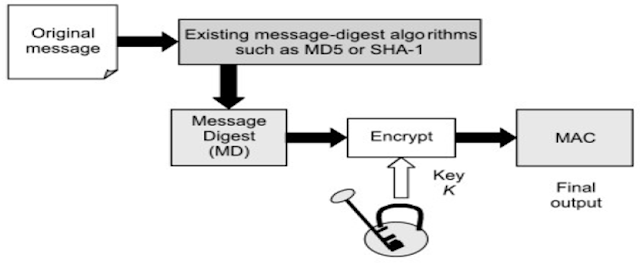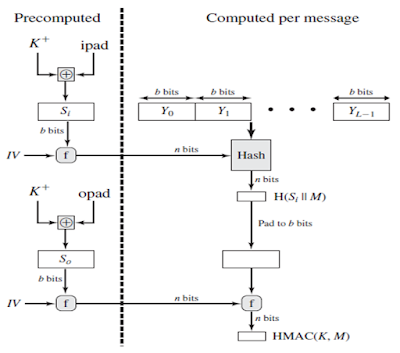## Tuesday, November 2, 2021

### HMAC | MAC based on Hash Function | HMAC Structure | HMAC Impelmentation | HMAC Pros & Cons

HMAC Concept

HMAC stands for HASH Message Authentication Code (HMAC) is a specific technique for calculating a message authentication code (MAC) involving a combination of cryptographic hash function and a secret key cryptography.Figure: Block diagram of HMAC

HMAC Structure & ImplementationFigure: HMAC StructureFigure: HMAC Implementation

HMAC Algorithm

1. Append zeros to the left end of K to create a b-bit string K+ .
2. XOR (bitwise exclusive-OR) with ipad to produce the b-bit block Si.
3. Append M to Si.
4. Apply H to the stream generated in step 3.
5. XOR K+ with opad to produce the b-bit block S0.
6. Append the hash result from step 4 to S0.
7. Apply H to the stream generated in step 6 and output the result.

HMAC Algo Step by Step Explanation

Step – 1: Make the length of K+ equal to b.

If length of K+ < b: add 0 bit as required to the left of k.

If length of K+ = b: In this case, we do not take any action, and proceed to step 2.

If length of K+ > b: we need to trim k, for this, we pass K through the message-digest algorithm(H) selected for this particular instance of HMAC.

Step – 2: XOR K+ with ipad to produce Si.

XOR K+ (the output of step 1) and ipad to produce a variable called Si.

Equation, K+ ⊕ ipad = Si

Step – 3: Append original message M to Si

Take the original message (M) and simply append it to the end of Si.

Equation, [(K+ ⊕ ipad) || M] = Si || M

Step – 4: Apply Message-digest algorithm

The selected message-digest algorithm (e.g. MD5,SHA-l, etc.) is applied to the output of step 3.

Equation,  H[(K+ ⊕ ipad) || M] = H(Si || M)

Step – 5: XOR K+ with opad to produce So

XOR K+ (the output of step 1) with opad to produce a variable called as So.

Equation, K+ ⊕ opad = So

Step – 6: Append H to So

Append the message digest calculated in step 4 to the end of So.

Equation, (K+ ⊕ opad) || H [(K+ ⊕ ipad) || M] = So || H(Si || M)

Step – 7: Apply Message-digest algorithm

The selected message-digest algorithm (e.g., MD5, SHA-I, etc.) is applied to the output of step 6 (i.e. to the concatenation of So and H). Finally, we got MAC.

Equation, HMAC (K, M) = H [(K+ ⊕ opad) || H [(K+ ⊕ ipad) || M]]

Pros & Cons of HMAC

Pros:

HMAC is faster to compute and verify digital signatures because they use hash functions rather than public key.

HMACs can be used in some cases where the use of public key cryptography is prohibited.

HMACs are much smaller than digital signatures.

Cons:

Key exchange is main issue, so can’t prevent against replay of message attack.

HMAC cannot be used if the number of receivers is greater than one.

If multiple parties share the same symmetric key. How does a receiver know that the message was prepared and sent by the sender?